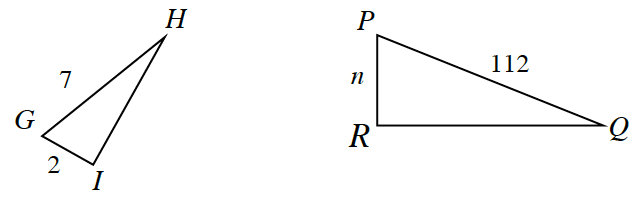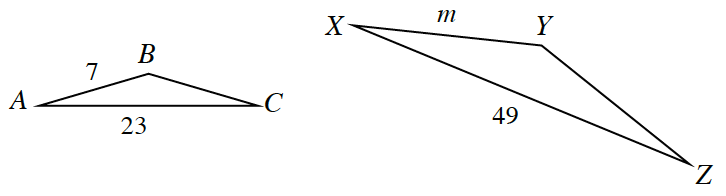### Home > INT2 > Chapter 2 > Lesson 2.3.3 > Problem2-108

2-108.

Solve for the missing lengths. Show all work.

1. $ΔGHI \sim ΔPQR$$\frac{2}{n} \ = \ \frac{7}{112}$

$32$

1. $ΔABC\simΔXYZ$$\frac{7}{m} \ = \ \frac{23}{49}$